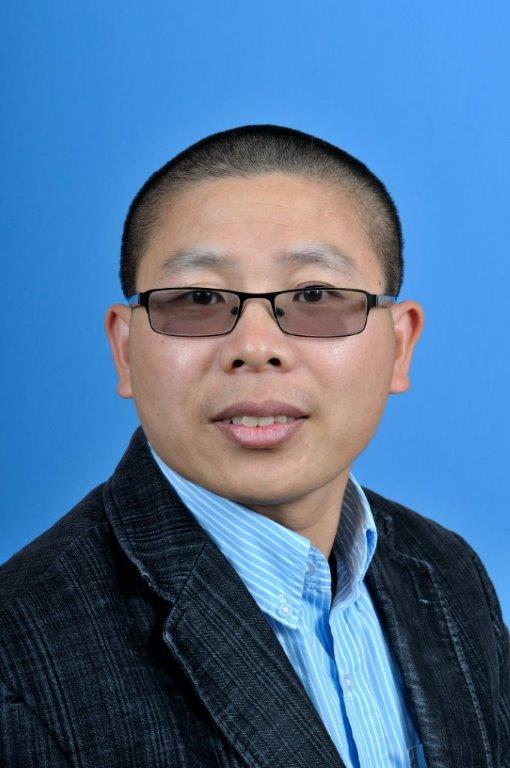# Dr. Xin TangProfessor in Mathematics

Office:  Science & Technology 424

Phone:  (910) 672-2206

Email: xtang@uncfsu.edu

Personal Homepage:

BIOGRAPHY

Graduated from Kansas State University in May 2006 with a Ph.D. degree in Mathematics. Joined Fayetteville State University in August 2006.

TEACHING

Math 121-Introduction to College Algebra
Math 123-College Algebra
Math 126-Quantative Reasoning
Math 129-PreCalculus I
Math 130-PreCalculus II
Math 131-Algebra and Trigonometry
Math 140-Applied Calculus
Math 142-Calculus with Analytical Geometry I
Math 150-Discrete Mathematics I
Math 241-Calculus with Analytic Geometry II
Math 242-Calculus with Analytic Geometry III
Math 251-Linear Algebra
Math 361-Introduction to Modern Algebra I
Math 362-Introduction to Modern Algebra II
Math 472-Theory of Numbers
Math 481-Introduction to Topology

RESEARCH INTERESTS

Noncommutative Algebraic Geometry and Representation Theory
Derivations and Endomorphisms for Quantum Algebras
Hopf Actions and Cancellation Problems for Quantum Algebras

SELECTED PUBLICATIONS

Tang, Xin Down-up algebras over a polynomial base ring K[t1,...,tn]. J. Algebra Appl. 15 (2016), no. 2,1650024, 16 pp.
Tang, Xin Algebra endomorphisms and derivations of some localized down-up algebras. J. Algebra Appl.14 (2015), no. 3, 1550034, 14 pp.
Tang, Xin Derivations of the two-parameter quantized enveloping algebra U+r,s(B2). Comm. Algebra 41 (2013), no. 12, 4602-4621
Tang, Xin (Hopf) algebra automorphisms of the Hopf algebra Uˇ≥0r,s(sl3). Comm. Algebra 41 (2013), no. 8, 2996-3012.
Tang, Xin Mad subalgebras and Lie subalgebras of an enveloping algebra. Bull. Aust. Math. Soc. 82 (2010), no. 3, 401-423
Tang, Xin Ringel-Hall algebras and two-parameter quantized enveloping algebras. Pacific J. Math. 247 (2010), no. 1, 213-240.
Xu, Yun GeTang, Xin Hochschild (co)homology of Galois coverings of Grassmann algebras. Acta Math. Sin. (Engl. Ser.) 25 (2009), no. 10, 1693-1702.
Tang, XinXu, Yunge On representations of quantum groups Uq(fm(K,H)). Bull. Aust. Math. Soc. 78 (2008), no. 2, 261-284.
Tang, Xin Construct irreducible representations of quantum groups Uq(fm(K)). Front. Math. China (2008), no. 3, 371-397.
Tang, Xin On irreducible weight representations of a new deformation Uq(sl2) of U(sl2). J. Gen. Lie Theory Appl. (2007), no. 2, 97-105.
Tang, Xin On Whittaker modules over a class of algebras similar to U(sl2). Front. Math. China (2007), no. 1, 127-142.
Tang, XinWu, Qiuxian Nakayama algebras and syzygy. Beijing Shifan Daxue Xuebao 35 (1999), no. 4,443-446.